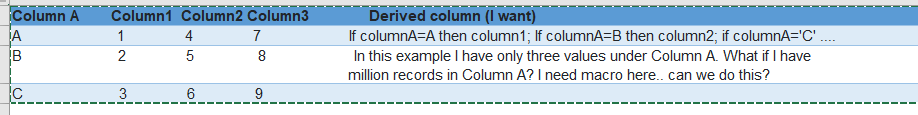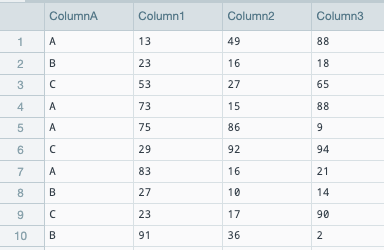••Hello Umasankar.

Datameer do not have Macros in the way they implemented in e.g MS Excel. However, perhaps your requirements could be fulfilled with available functionality.

If you will describe: a) the problem you are trying to resolve, b) the initial dataset, and c) required data transformation details, I could try to point you to appropriate methods.

••Hello Umasankar.

• Is the requirement to implement a unique condition for every distinct value at column A?
• How many distinct THEN clauses do you have?

Perhaps you could briefly describe the use-case you are working on, e.g. what exactly are you trying to calculate/determine?

•Hi Umasankar,

I have an additional question for you.  How would the tool know what column to resolve based on the value in your "Column A" if it's not specified?

If we expand your example:  A resolves to 1, B resolves to 2, C resolves to 3.  What if the next value in Column A is E?  Would that map to Column 4 or Column 5?  Likewise, what if the next value after E is D?  What happens then?

How many distinct values are in Column A?

•Let say I have 3 distinct values in Column A. I know the values, based on the values I have to pick specific column  (Either column1, 2 or 3) and pick relevant values under that specific column

•Hi Umasankar,

This can be achieved with the CASE statement or a nested IF statement.

You can see a full working example with sample data within the CASE documentation.

Simply replace the <return value 1> with the pointer for your column.

Similarly you can find a working example with sample data within the IF documentation.

I'd suggest CASE since it's more narrow and your example seems to fit the criteria for usage.

•Hello Umasankar.
Let's take the following source data as an example.The following set of nested IF functions will return in the Result column:

• Value from Column1 if the value in ColumnA is "A".
• Value from Column2 if the value in ColumnA is "B".
• Value from Column3 if the value in ColumnA is "C".
• Empty value if the value in ColumnA is not "A", "B" or "C".

IF(#ColumnA=="A";#Column1;IF(#ColumnA=="B";#Column2;IF(#ColumnA=="C";#Column3;null)))In case ColumnA contains only "A", "B" or "C you could drop the last check only simplyfy the formula to:

IF(#ColumnA=="A";#Column1;IF(#ColumnA=="B";#Column2;#Column3))

I hope this approach will be helpful.